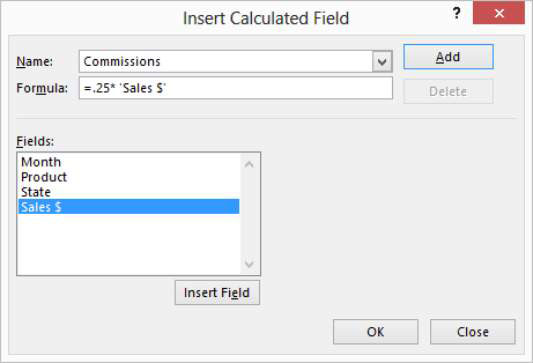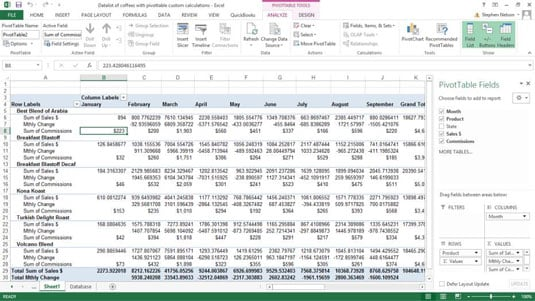##### Excel 2003Â For Dummies Quick ReferenceExcel supplies an opportunity for calculating values inside a pivot table. You can add calculated fields and items to a table. Adding a calculated field enables you to insert a new row or column into a pivot table and then fill the new row or column with a formula.

For example, you see that it reports on sales both by product and month. What if you want to add the commissions expense that you incurred on these sales?

Suppose for the sake of illustration that your network of independent sales representatives earns a 25 percent commission on coffee sales. This commission expense doesn't appear in the data list, so you can't retrieve the information from that source. However, because you know how to calculate the commissions expense, you can easily add the commissions expense to the pivot table by using a calculated field.

To add a calculated field to a pivot table, take the following steps:

1. Identify the pivot table by clicking any cell in that pivot table.

2. Tell Excel that you want to add a calculated field.

Click the Analyze ribbon’s Fields, Items & Sets command, and then choose Calculated Field from the Formulas menu. Excel displays the Insert Calculated Field dialog box.

In Excel 2007and Excel 2010, you choose the PivotTable Tools Option tab’s Formulas command and then choose Calculated Field from the Formulas menu.3. In the Name text box, name the new row or column that you want to show the calculated field.

For example, if you want to add a row that shows commissions expense, you might name the new field Commissions.

4. Write the formula in the Formula text box.

Calculated field formulas work the same way as formulas for regular cells:

1. Begin the formula by typing the equal (=) sign.

2. Enter the operator and operands that make up the formula.

If you want to calculate commissions and commissions equal 25 percent of sales, enter =.25*.

3. The Fields box lists all the possible fields that can be included in your formula. To include a choice from the Fields list, click the Sales \$ item in the Fields list box and then click the Insert Field button.

See how the Insert Calculated Field dialog box looks after you create a calculated field to show a 25 percent commissions expense.

5. Click OK.

Excel adds the calculated field to your pivot table.After you insert a calculated field, Excel adds the calculated field to the PivotTable field list. You can then pretty much work with the calculated item in the same way that you work with traditional items.